# Negative difference

Find four arithmetic progression members between 7 and -6.

a2 =  4.4
a3 =  1.8
a4 =  -0.8
a5 =  -3.4

### Step-by-step explanation: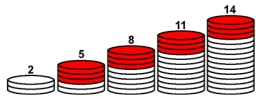Did you find an error or inaccuracy? Feel free to write us. Thank you!## Related math problems and questions:

• Insert 3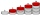Insert five arithmetic progression members between -7 and 3/2.
• Common difference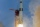The 4th term of an arithmetic progression is 6. If the sum of the 8th and 9th terms is -72, find the common difference.
• Difference AP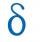Calculate the difference of arithmetic progression if the sum of its first 19 members Sn = 8075 and the first member is a1 = 20
• Sum of four numbers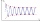The sum of four consecutive natural numbers is 114. Find them.
• Fifth memberDetermine the fifth member of the arithmetic progression, if the sum of the second and fifth members equal to 73, and difference d = 7.
• AP membersWhat is the value of x2, x3, x4, x5…of the terms (of arithmetic progression) when x1 = 8 and x6 = 20?
• Geometric progressionIn geometric progression, a1 = 7, q = 5. Find the condition for n to sum first n members is sn≤217.
• Arithmetic progression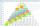In some AP applies: 5a2 + 7a5 = 90 s3 = 12 Find the first member a =? and difference d = ?
• What areWhat are the four terms between 4 and 19?
• Determine AP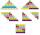Determine the difference of the arithmetic progression if a3 = 7, and a4 + a5 = 71
• Find the meanFind the number between 13 and 29.
• Find the 19Find the 1st term of the GP ___, -6, 18, -54.
• Five element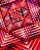The geometric sequence is given by quotient q = 1/2 and the sum of the first six members S6 = 63. Find the fifth element a5.
• Harmonic seriesInsert four members between 5/3 and 5/11 to form harmonic series (means).
• GP - three membersThe second and third of a geometric progression are 24 and 12(c+1), respectively, given that the sum of the first three terms of progression is 76. determine the value of c.
• Simple sequence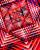Continue with this series of numbers: 1792,448, 112, _, _
• GP membersThe geometric sequence has 10 members. The last two members are 2 and -1. Which member is -1/16?